Function Repository Resource:

# Fibbinary

Get the fibbinary sequence

Contributed by: Wolfram Staff, based on work by Jörg Arndt, Robert G. Wilson and Eric W. Weisstein
 ResourceFunction["Fibbinary"][n] gives the nth fibbinary number. ResourceFunction["Fibbinary"][{n}] gives a list of fibbinary numbers less than or equal to n.

## Details

Fibbinary numbers are the positive integers whose binary representation contains no consecutive ones.

## Examples

### Basic Examples (3)

First one hundred fibbinaries:

 In:=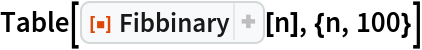Out=Fibbinaries less than or equal to 50, represented in base 2:

 In:=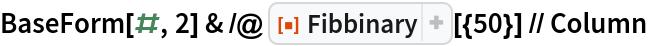Out=Plot of fibbinaries less than 150 in base 2:

 In:=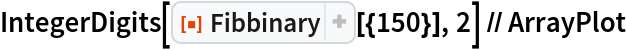Out=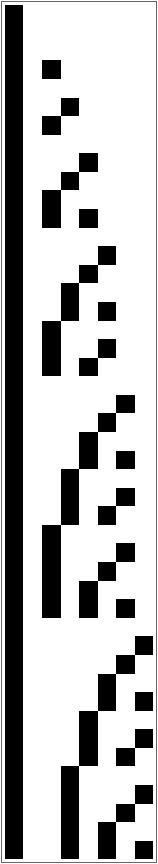### Properties and Relations (2)

Fibbinaries are related to the Zeckendorf representation:

 In:=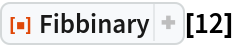Out=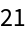In:=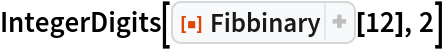Out=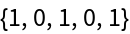In:=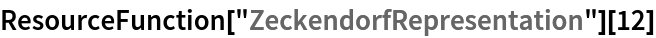Out=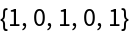Adding the following integers gives 12:

 In:=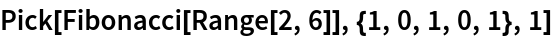Out=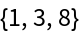In:=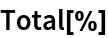Out=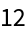## Requirements

Wolfram Language 11.3 (March 2018) or above

## Version History

• 1.0.2 – 04 October 2021